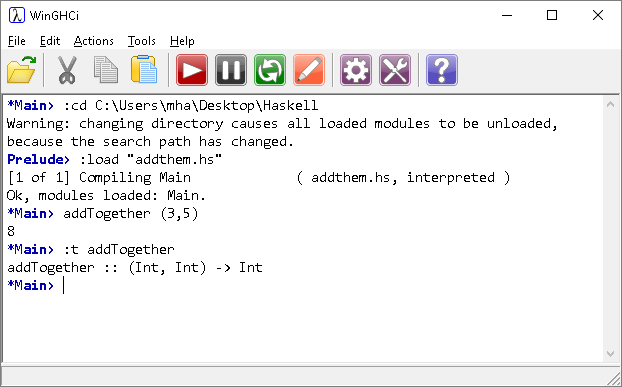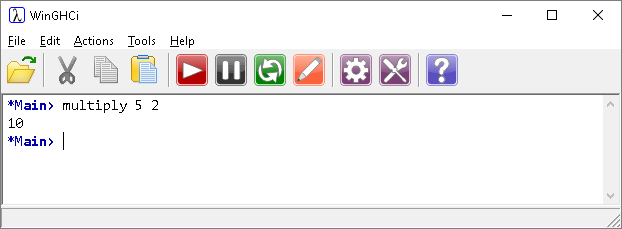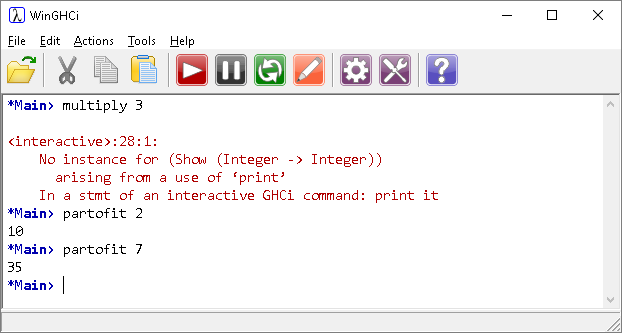# Introduction To Haskell Partial Function Application

## Introduction

In Haskell, functions are considered first-class objects as noted in the AQA specification.

Know that a function is a first-class object in functional programming languages and in imperative programming languages that support such objects. This means that it can be an argument to another function as well as the result of a function call.

First-class objects in any programming language are those elements that can,

• appear in expressions,
• be assigned to a variable,
• be assigned as arguments,
• be returned in function calls.

Consider the following function,

```addTogether :: Int -> Int -> Int
addTogether a b = a + b
```

We can apply the function by supplying two arguments,We cannot, however, supply anything other than 2 arguments when applying the function.Now, let's define a multiplication function,

```multiply :: Integer -> Integer->Integer
multiply x y = x * y
```

The first line defining the type is different from what we have used before. The 'arrow' (->) means an argument being applied to a function. This type definition means the multiply takes an integer and returns a function which takes an integer and returns an integer.What is happening here is that the function is being partially applied to the 5 and the resulting function is then applied to the 2.

Broken down a little, it can be easier to see what is happening.

```multiply :: Integer -> Integer->Integer
multiply x y = x * y

partofit = multiply 5
```

The function, partofit contains a partial application of the multiply function.Notice that the multiply function still won't accept a single argument. The new function does.

Since functions can accept and return functions, we can chain function applications together to achieve the effect we are looking for,